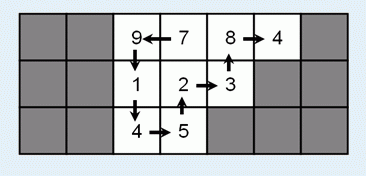# #2477. Biggest Number

### Description

You have a maze with obstacles and non-zero digits in it:You can start from any square, walk in the maze, and finally stop at some square. Each step, you may only walk into one of the four neighbouring squares (up, down, left, right) and you cannot walk into obstacles or walk into a square more than once. When you finish, you can get a number by writing down the digits you encounter in the same order as you meet them. For example, you can get numbers 9784, 4832145 etc. The biggest number you can get is 791452384, shown in the picture above.

### Input

There will be at most 25 test cases. Each test begins with two integers R and C (2<=R,C<=15, R*C<=30), the number of rows and columns of the maze. The next R rows represent the maze. Each line contains exactly C characters (without leading or trailing spaces), each of them will be either '#' or one of the nine non-zero digits. There will be at least one non-obstacle squares (i.e. squares with a non-zero digit in it) in the maze. The input is terminated by a test case with R=C=0, you should not process it.

### Output

For each test case, print the biggest number you can find, on a single line.

3 7
##9784#
##123##
##45###
0 0

791452384